Select Page

# Process Costing

Previous Lesson: Job Order Costing

Next Course: Cost Volume Profit Analysis

Process costing is a method of costing used to ascertain the cost of production of each process, operation or stage of manufacture where processes are carried in having one or more of the following features:

1. The output of one process becomes the input to the next process
2. The continuous nature of Homogeneous production
3. There is often a loss in process due to spoilage, shrinkage, wastage so on
4. Output from production may be a single product, but there may also be a by-product (or byproducts) and/or joint products

Process costing is a costing method used where it is not possible to identify separate units of production, or jobs, usually because of the continuous nature of the production processes. It is common to identify process costing with continuous production such as the following:

• Oil refining
• Foods and drinks
• Paper
• Chemicals

Difference between Job Order Costing and Process Costing

However, there are some important differences between job order and process costing as described below:## Process Costing (Cost of Production Report)

In process costing Cost of Production Report also called Process Cost Sheet is the key document. At the end of costing period, generally a month, a Cost of Production Report is prepared. It summarizes the data of quantity produced and cost incurred by each producing department. It also serves as a source document for passing accounting entries at the end of costing period. A cost of production report shows:

• Total unit costs transferred to it from a preceding department
• Unit cost added by the department
• Total and unit costs accumulated to the end of operations in the department.
• The cost of the beginning and ending work in process inventories
• Cost transferred to a succeeding department or to a finished goods storeroom

Cost of production report is divided into five sections. Each section is meant to provide specific information. A brief description of these sections is presented below:

1. Quantity Schedule
2. Cost Charged to the Department
3. Equivalent units produced
4. Cost Per Unit
5. Cost Accounted for as Follows

## Example # 1:

A manufacturing company makes a single production in one department; you are required to make a Cost of Production Report (CPR) from the following data:

Cost Data

Material Cost                 Rs. 24,500

Labor Cost                      Rs. 29,450

Production Data

Unit started for production   50,000 Units

Unit completed                      45,000 Units

Unit in process                        5,000 Units

At the end of month Raw material 100% completed and Labor and FOH 50%.

### Solution:

#### Quantity Schedule

The first section Quantity Schedule contains input and output data in terms of quantities. The information is presented in the following order.

 Quantity Schedule: Units started in process 50,000 ====== Units completed and transferred to next department 45,000 Units still in process (All materials – 50% Labor and FOH) 5,000 50,000======

#### Cost Charged to the Department

This section contains direct materials, direct labor and factory overhead added by the department:

 Cost Charged to the Department: Materials Rs. 24,500 Labor 29,450 Factory Overhead (FOH) 28,500——- Cost Charged to the Department Rs. 82,450 ======

#### Equivalent Units Produced

In order to arrive at cost per unit of output, total of each cost element is divided by the number of units produced. For this purpose, where at the end of costing period, there are some partially completed units in process, these units must be stated in terms of equivalent completed units. These equivalent units are added to units completed by the department to arrive at equivalent production. Then total cost is divided by this equivalent production figure to calculate unit cost.

 Equivalent Units Produced: Materials (45,000) + (5,000*100%) 50,000 Units Labor (45,000) + (5,000*50%) 47,500 Units Factory Overhead (45,000) + (5,000*50%) 47,500 Units===========

#### Cost per Unit

The unit cost of the equivalent units for a given period is calculated as follows:Cost per Unit: Materials (24,500 / 50,000) 0.49 per Units Labor (29,450 / 47,500) 0.62 per Units Factory Overhead (28,200 / 47,500)     Total per unit cost 0.60 per Unit     1.71 per Unit

#### Cost Accounted for as Follows

This section shows total cost for which the departments are accountable:

 Cost Accounted for as Follows: Transferred to next department (45,000 × Rs. 1.71) Rs. 76,950 Work in process – ending inventory: Materials (5,000 × 100% × Rs. 0.49) Rs. 2,450 Labor (5,000 × 50% × Rs. 0.62) 1,550 Factory Overhead (5,000 × 50% × Rs. 0.60) 1,500 5,500———- Total cost accounted for Rs. 82,450 ========

>> Practice Process Costing MCQs

## One Department Case

This case assume that company has a one department

## Example # 2:

Mini Soap Manufacturing units started to incurring cost in first department for 1,000 soaps. At the end of the week 600 soaps were completed and 400 incomplete. 100% of direct material had been incurred. But 75% conversion cost was yet incurred on the incomplete work. Detail of cost incurred by the department as follows:

Direct Material                     Rs. 500

Direct Labor                         Rs. 225

FOH                                       Rs.135

Required: Prepare Process Costing Report  (Cost of Production Report) at the end of March, 2018.

### Solution:## Example # 3:

Heera Manufacturing Company uses process costing to manufactures a product. Production made and manufacturing costs incurred in the first department during the month of October, 2017 are given below:

10,000 units were started in process out of which 9,400 units were transferred to next department and remaining 600 units were 1/2 complete as to materials, labor and overhead. Direct materials Rs. 19,400, direct labor Rs. 24,250 and factory overhead Rs. 4,850 was charged to production.

Required: Cost of production report for the month.

### Solution: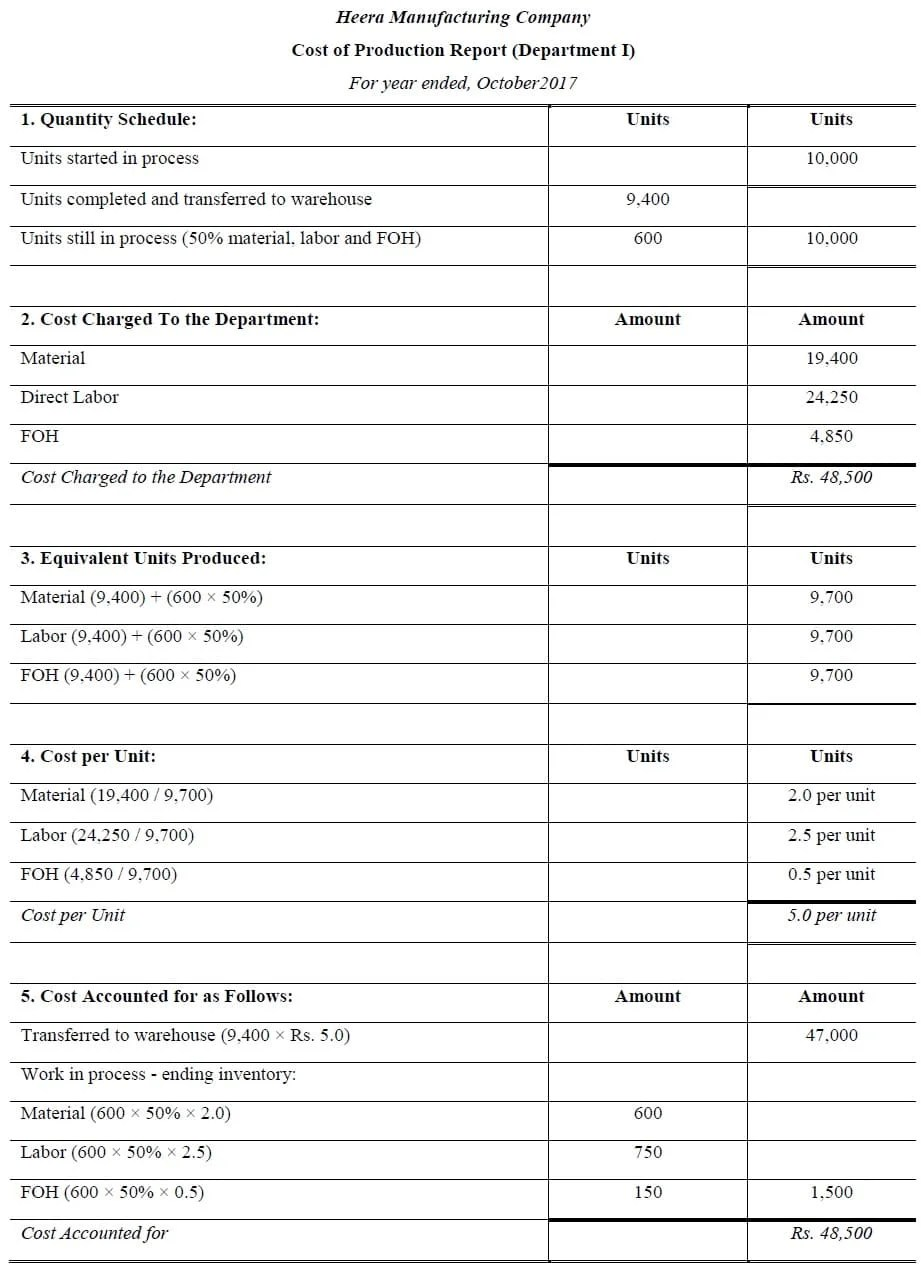## Example # 4:

Production and cost data of first production department of Excellent Manufacturing Company for the month of January, 2018 are as follow:

Units started in process were 5,000. Units completed and transferred to second department were 4,500. Remaining units were in process estimated to be 50%, 40%, 60% completed as to materials, labor and factory overhead respectively. Costs of materials, labor and overhead were Rs. 49,875, Rs. 59,925 and Rs. 40,032 respectively.

Required: Cost of production report

### Solution:>> Practice Process Costing Problems and Solutions

## More than One Department Case

In this case it is assume that company has more than one department for processing

## Example # 5:

Mini Soap Manufacturing units started to incurring cost in first department for 1,000 soaps. At the end of the week 600 soaps were completed and 400 incomplete. 100% of direct material had been incurred. But 75% conversion cost was yet incurred on the incomplete work. Detail of cost incurred by the department I as follows:

Direct Material                                                     Rs. 500

Direct Labor                                                         Rs. 225

FOH                                                                       Rs.135

Mini Soap Manufacturing unit completed and transferred out 600 soaps to department II. In department II 500 soaps completed and transferred out. Units which were still in process 100 (100% material, Conversion cost 60%). Cost received from preceding department Rs. 540

Following costs were incurred by department II

Direct Material                                                     Rs. 150

Direct Labor                                                         Rs. 112

FOH                                                                       Rs. 168

Required: Cost of Production Report at the end of March, 2018 for department I and II.

### Solution: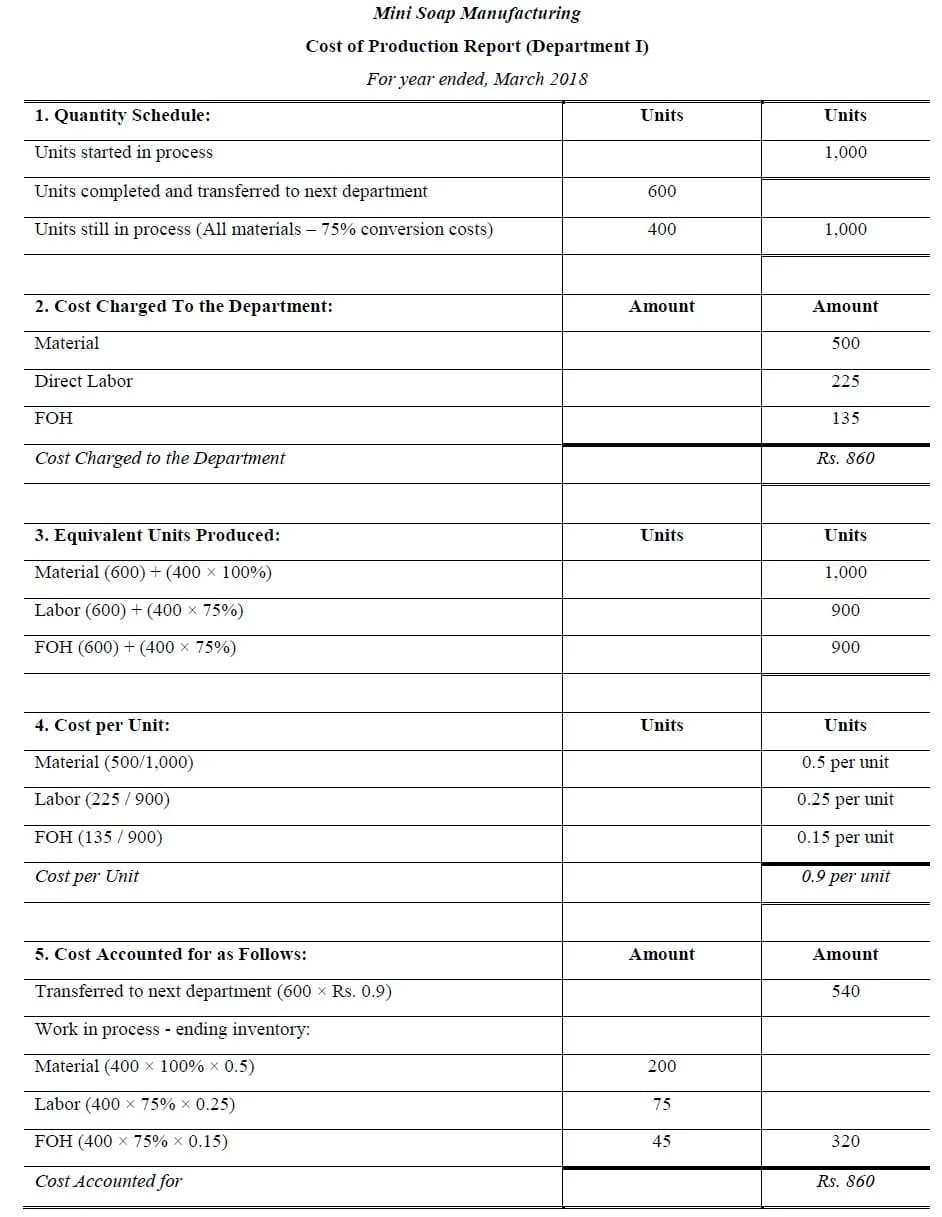## Example # 6:

Nadeem manufacturing company produces products. The processing of this product is to be done in three departments known as department A, B and C. from department A the work passed sequentially through department B and C, emerging as finished product. The cost transactions of the company are shown in the following journal entries:The quantity of production statement for this period appears as follows: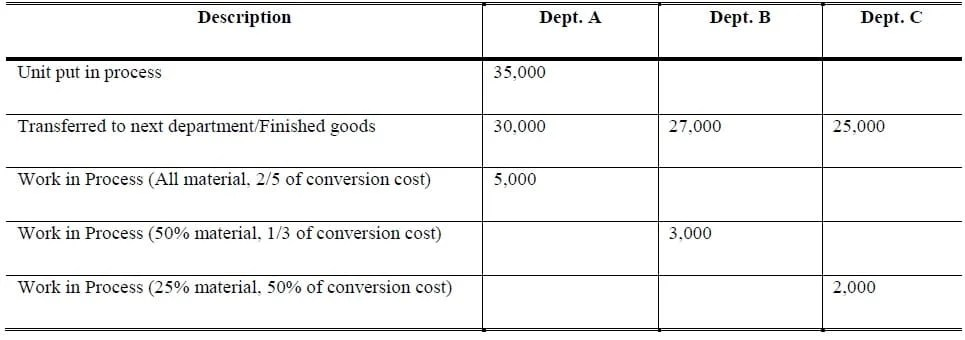Requirement: Prepare cost of Production Report

>> Practice Process Costing MCQs

### Solution: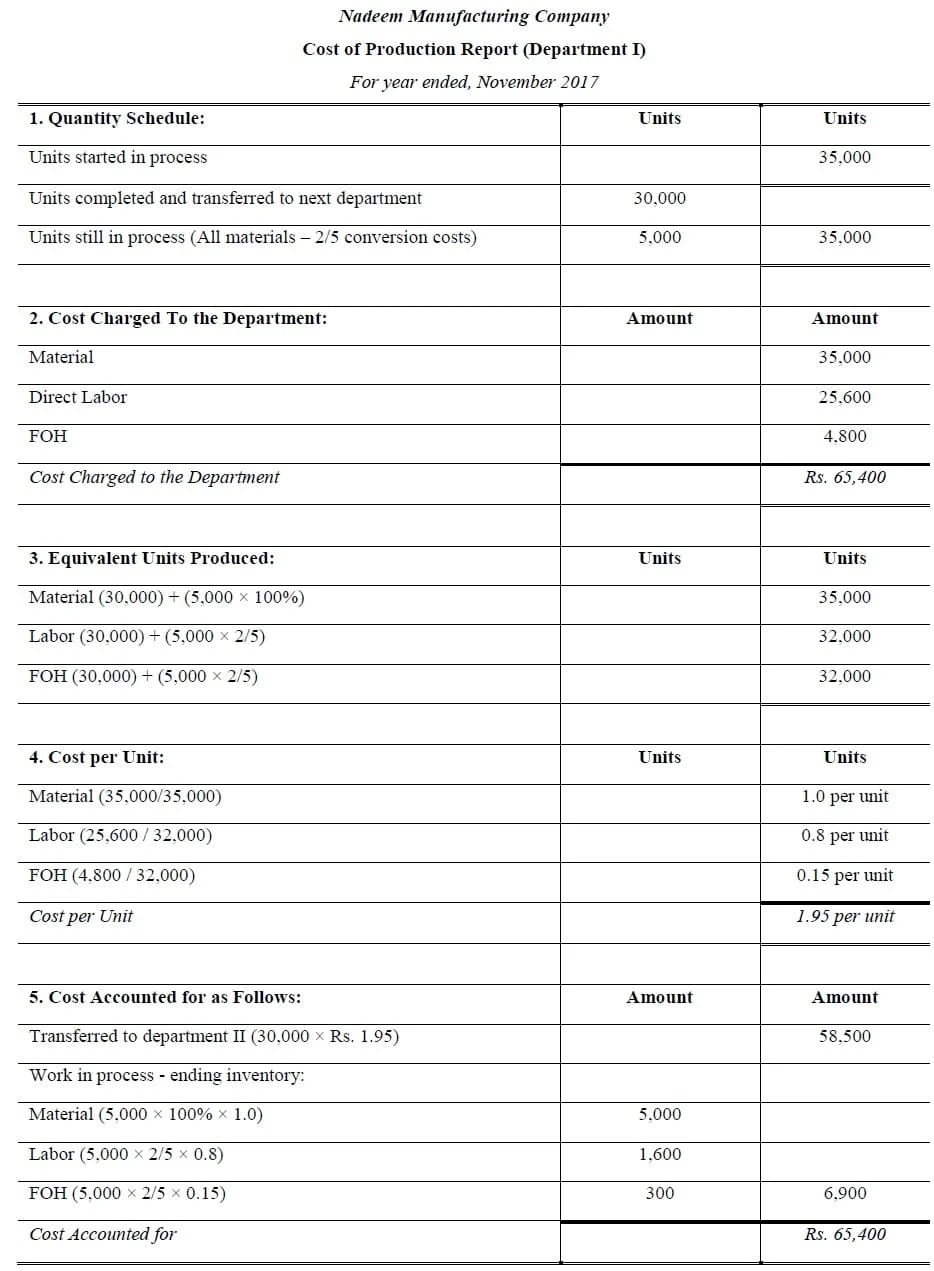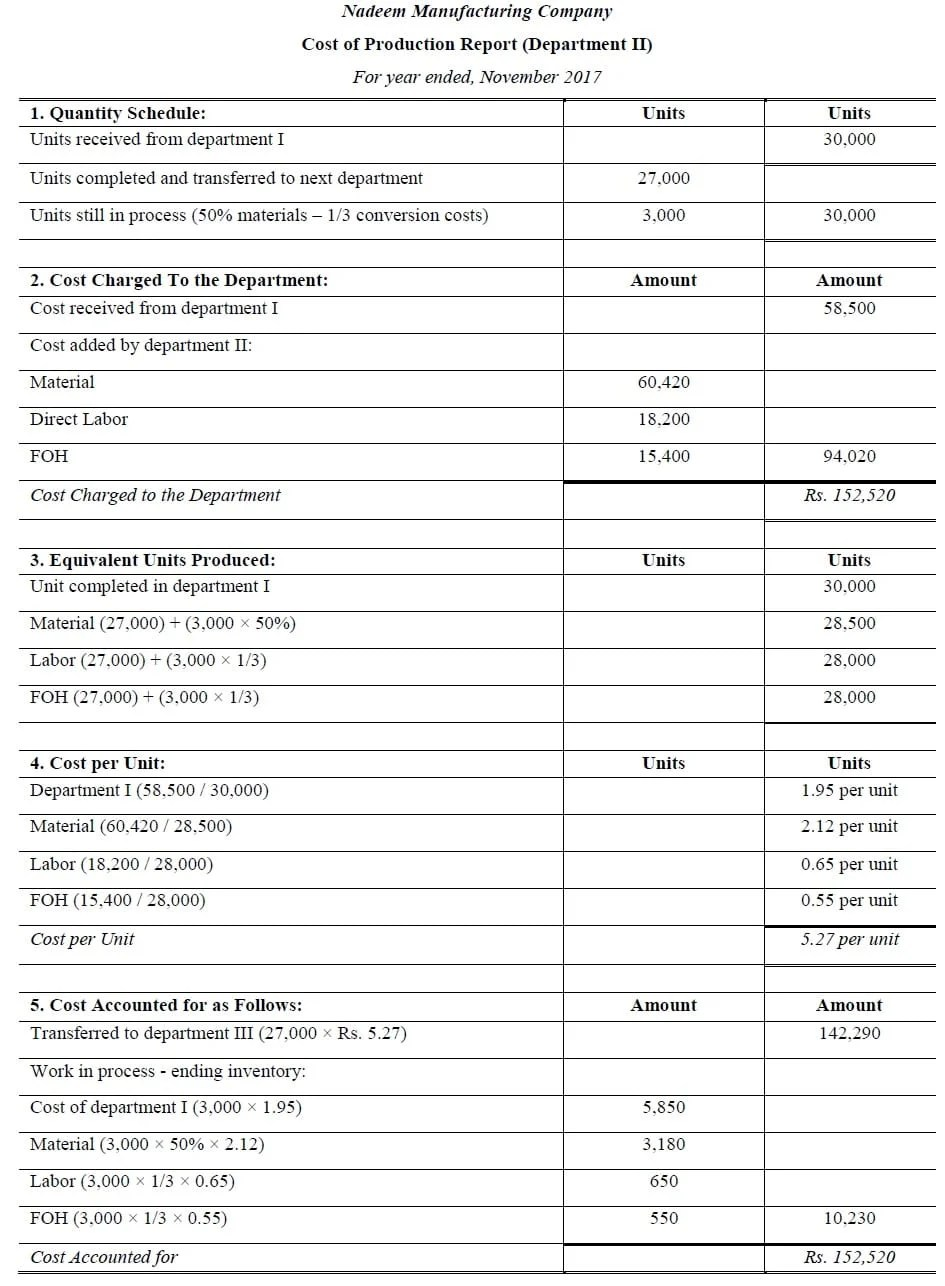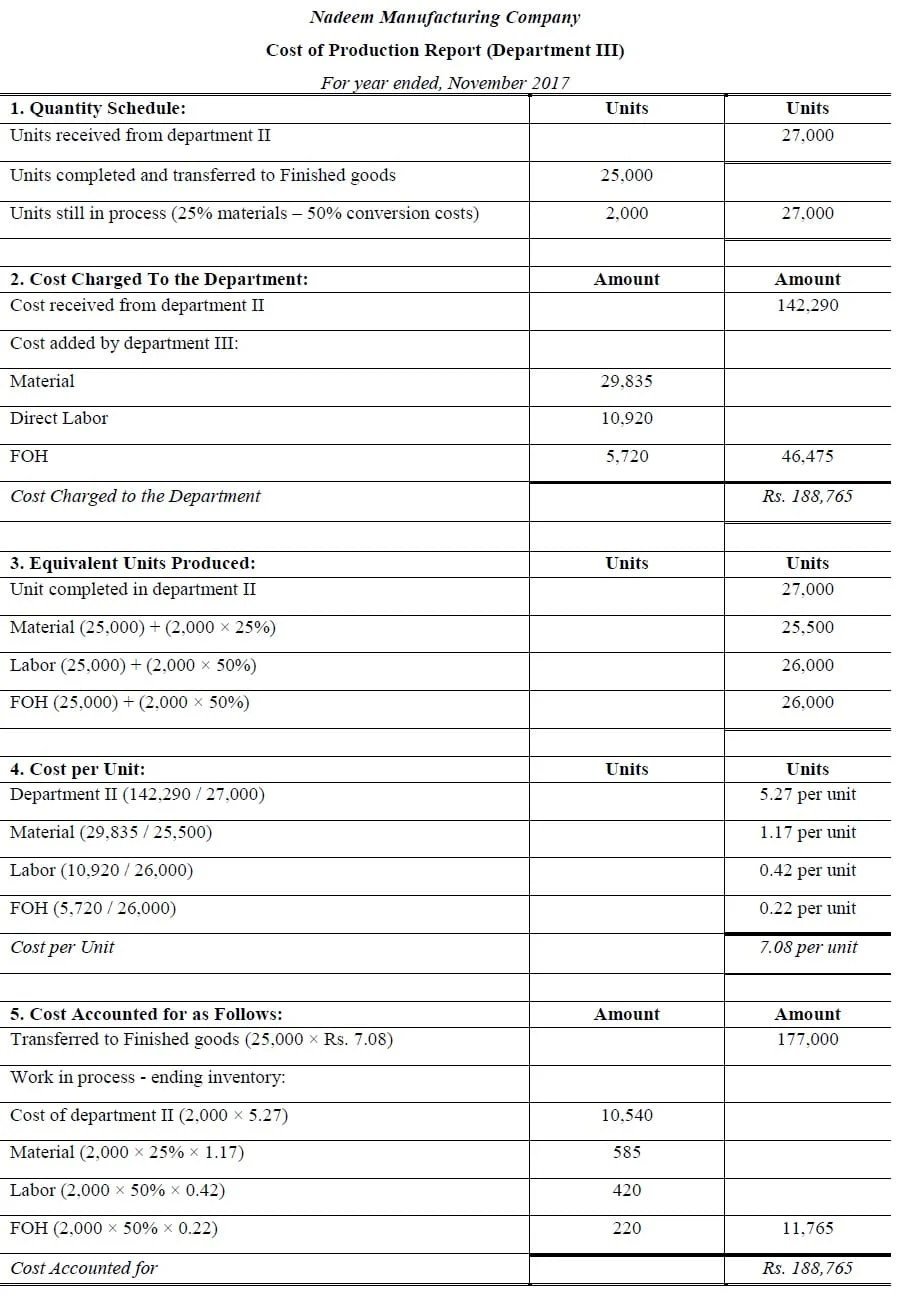>> Practice Process Costing Quiz 1 and Quiz 2.

## References

Mukharji, A., & Hanif, M. (2003). Financial Accounting (Vol. 1). New Delhi: Tata McGraw-Hill Publishing Co.

Narayanswami, R. (2008). Financial Accounting: A Managerial Perspective. (3rd, Ed.) New Delhi: Prentice Hall of India.

Ramchandran, N., & Kakani, R. K. (2007). Financial Accounting for Management. (2nd, Ed.) New Delhi: Tata McGraw Hill.

## 1 Comment

1. i need the solution of process costing MCQs